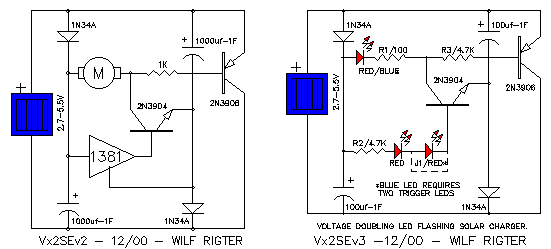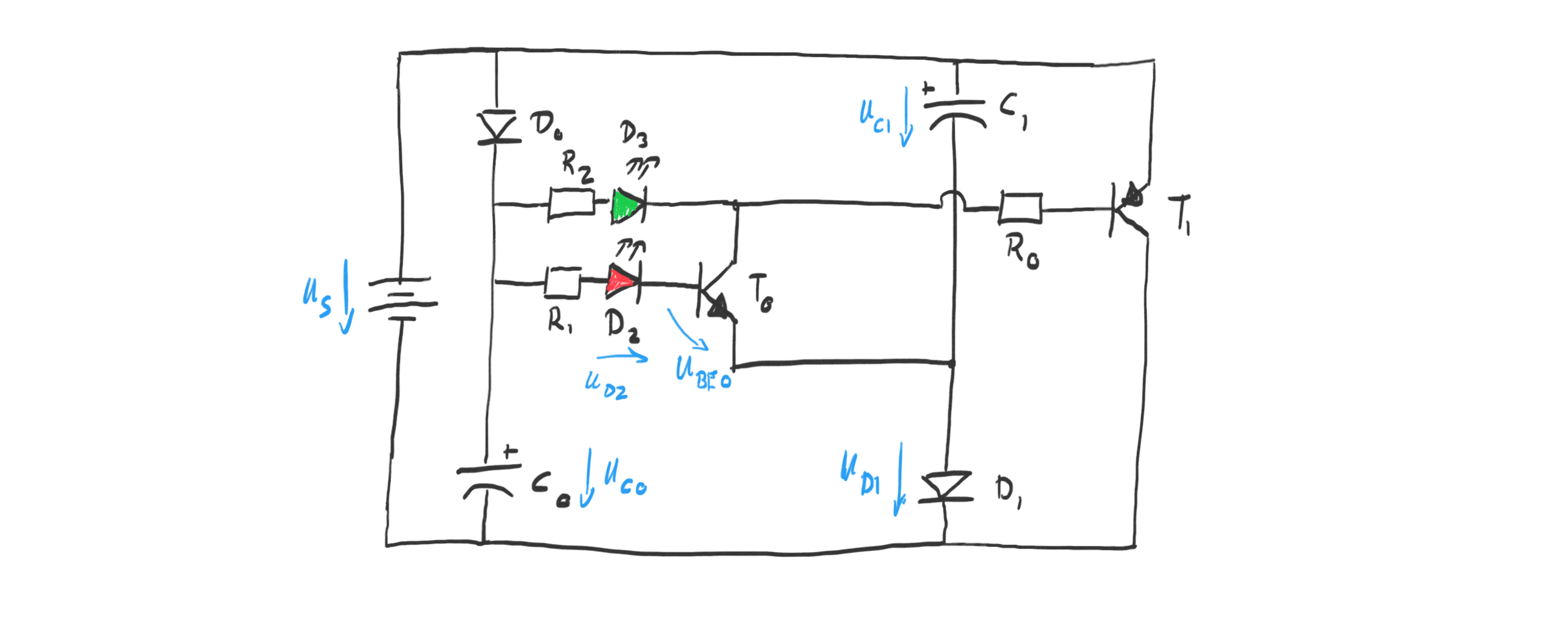# Julian Straub

## creations and thoughts

Building the pretty straight forward light harvester described in a previous post, I learned that that design is somewhat passive: the harvester just charges the whole day and then discharges for 1-2h (depending on the capacitor) once it gets dark.

To explore a more active harvester that periodically emits energy whenever the capacitor is charged, I reimplemented the VxSE3 solar engine by WLF Rigter:Reproduced here with my specific adaptations:

• used a green LED with forward voltage of 3.2V
• a solar panel with 3V output
• $$10\mu F$$ storage capacitorsThe circuit above is pretty clear for the charging cycle: the capacitors are charged in parallel each through its own diode. I used Schottky diodes with a forward drop of 0.4V. Given the 3V solar cell this means they will each be charged to about $$U_{c0} = U_{c1} = 2.6V$$ .

Once the capacitors reach their full charge the transistor $T0$ goes into conduction. We can see that by looking at the voltage $U{BE0}$:

$$U{BE0} = U{c0} - U{d1} - U{d2} - U{R1} = 2.6V - 1.8V - U{R1} = 0.8V - U_{R1}$$

which is $>0.7V$ since the resistor just acts to limit the current into the transistor.

With $T_0$ saturated and in conduction it is instructive to look at this re-drawing of the circuit to understand the voltage doubling feature of this circuit:As soon as $T_0$ is in conduction, the output of the green LED $D_3$ is pulled to the potential of the lower capacitor $C_1$. The base of the pnp transistor $T_1$ is pulled to the same potential as well. The saturates $T_1$ as can be seen from the base-emitter voltage of $T_1$:

$$U{BE1} = -U{R0} - U{c1} + U{BE0} \approx -2.4V -U_{R0} < -0.7V$$

The saturated npn transistor $T_1$ then connects the two capacitors leading to an approximate doubling of the input voltage. More precisely, the following voltage is applied over the green LED and its resistor:

$$U{D3} + U{R2} = U{c0} + U{c1} - U{CE1} - U{CE0} \approx 2 U_{c0} - 0.4V = 4.8V$$

The resistor $R_2$ limits the discharge current through the green LED $D_3$ as usual, in the most simple LED circuit. As the capacitors discharge the voltage eventually drops too low to keep transistor $T_0$ in conduction and the circuit resets to charging mode.